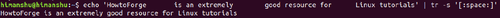Launch Apache Spark™ clusters in minutes with 14 days of Azure Databricks Units for free.# Linux tr Command Tutorial for Beginners (with Examples)

Depending on the kind of work you do on the command line in Linux, you may want a utility that can act as a Swiss army knife of quick text editing. Gladly, there exists a tool dubbed tr, which qualifies for this role. In this tutorial, we will discuss the basics of tr using some easy to understand examples.

But before we do that, it's worth mentioning that all examples in this article have been tested on an Ubuntu 18.04 LTS machine.

## Linux tr command

Here's how the tool's man page explains it:

``Translate, squeeze, and/or delete characters from standard input, writing to standard output.``

And following is its syntax:

``tr [OPTION]... SET1 [SET2]``

here's what SET means:

``SETs are specified as strings of characters.  Most represent themselves.  Interpreted sequences are:       \NNN   character with octal value NNN (1 to 3 octal digits)       \\     backslash       \a     audible BEL       \b     backspace       \f     form feed       \n     new line       \r     return       \t     horizontal tab       \v     vertical tab``

Following are some Q&A styled examples that should give you a better idea on how the tr command works.

## Q1. How to convert lower case to upper case using tr?

Suppose you want to convert the sentence "linux tutorial on howtoforge" to uppercase, then here's how you can do this using tr.

``echo 'linux tutorial on howtoforge' | tr "[:lower:]" "[:upper:]"``

The above command produced the following output on my system:

``LINUX TUTORIAL ON HOWTOFORGE``

## Q2. How to strip extra spaces using tr?

Suppose you have a line like: "HowtoForge       is an extremely        good resource for      Linux tutorials". And the requirement is to strip extra spaces from this line.

Here's how you can use tr to do this:

``echo 'HowtoForge       is an extremely        good resource for      Linux tutorials' | tr -s '[:space:]'``

Here's the output:

``HowtoForge is an extremely good resource for Linux tutorials``## Q3. How to delete text using tr?

Suppose you want to delete the hyphens from the following line: "HowtoForge -- is -- an -- extremely -- good -- resource -- for -- Linux -- tutorials." Then here's how you can do this using tr.

``echo 'HowtoForge -- is -- an -- extremely -- good -- resource -- for -- Linux -- tutorials' | tr -d '-'``

Following is the output it produces:

``HowtoForge  is  an  extremely  good  resource  for  Linux  tutorials``

## Q4. How to replace characters using tr?

In the previous section, suppose the requirement was to replace hyphens with, let's say, dots. Then here's how you can do that using tr.

``echo 'HowtoForge -- is -- an -- extremely -- good -- resource -- for -- Linux -- tutorials' | tr '-' '.'``

Following is the output it produced:

``HowtoForge .. is .. an .. extremely .. good .. resource .. for .. Linux .. tutorials``

## Conclusion

So you can see the tr command is an extremely helpful tool when it comes to editing text. We have discussed some main options here, but the utility offers many other command line options as well. First try these, and once you've got a good idea about what we've discussed here, then you can learn more about tr by heading to its man page.view as pdf |print